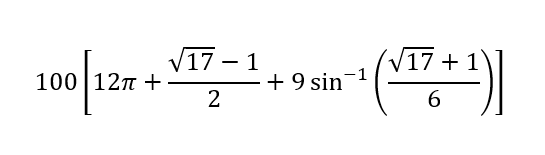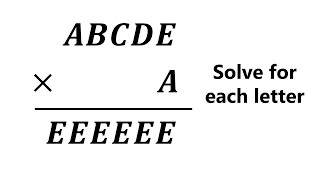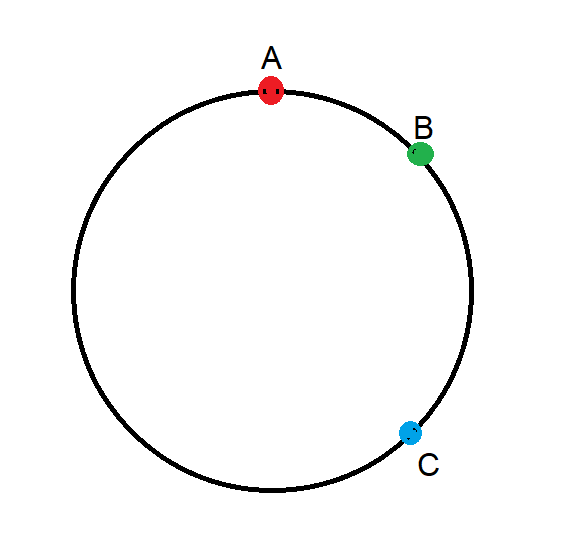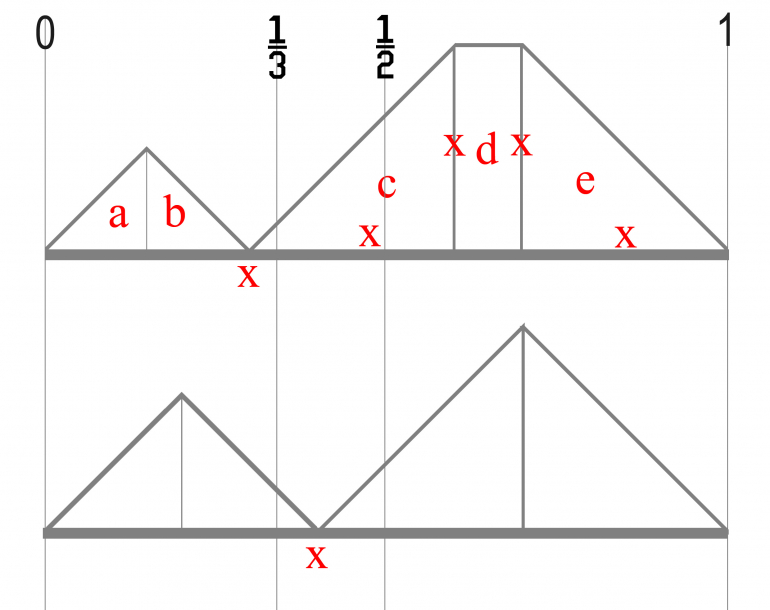## Poll

 I love math!20 votes (46.51%) Math is great.14 votes (32.55%) My religion is mathology.6 votes (13.95%) Women didn't speak to me until I was 30.2 votes (4.65%) Total eclipse reminder -- 04/08/202412 votes (27.9%) I steal cutlery from restaurants.3 votes (6.97%) I should just say what's on my mind.6 votes (13.95%) Who makes up these awful names for pandas?5 votes (11.62%) I like to touch my face.12 votes (27.9%) Pork chops and apple sauce.9 votes (20.93%)

43 members have voted

ThatDonGuyJoined: Jun 22, 2011
• Posts: 5680
July 20th, 2022 at 5:01:14 PM permalink
I can show how to calculate ChesterDog's value.
The area is symmetrical with respect to line BC (i.e. the line that goes through B and C), so only half of it needs to be calculated, and that value doubled.
Half of the area can be divided into three sections:

1 - the red area, which is 3/8 of a circle of radius 40; this is area 3/8 x PI x 40^2 = 600 PI (it is 3/8 because 1/4 of the circle centered at A is taken up by the white square, and half of the remainder is 3/8)

2 - the yellow triangle, which, as I have already shown, is 25 (sqrt(17) - 1)

3 - the blue area; note it is a portion of a circle of radius 30, so the area is 900 PI x the measure (in radians) of the angle at A divided by 2 PI = 450 x the measure of the angle at A.
Let t be the measure of angle CAD; the measure of the angle at A is (PI/2 - t) radians.
In the solution to the yellow triangle area, sin t = (sqrt(17) - 1) / 6; sin (PI/2 - t) = cos t = sqrt(1 - sin^2 t) = sqrt(1 - (sqrt(17) - 1)^2 / 36) = sqrt(1 - (18 - 2 sqrt(17)) / 36) = sqrt(18 + 2 sqrt(17)) / 6 = (sqrt(17) + 1) / 6, so the area = 450 sin^(-1) ((sqrt(17) + 1) / 6)

The area of the entire field covered by the goat = 2 (600 PI + 25 (sqrt(17) - 1) + 900 sin^(-1) ((sqrt(17) + 1) / 6)
= 1200 PI + 50 (sqrt(17) - 1) + 900 sin^(-1) ((sqrt(17) + 1) / 6)
= 100 (12 PI + (sqrt(17) - 1) / 2 + 9 sin^(-1) ((sqrt(17) + 1) / 6))
GialmereJoined: Nov 26, 2018
• Posts: 2589
July 20th, 2022 at 6:37:28 PM permalinkQuote: ChesterDog

I get about 4,847.07 square feet.

This is the result of the following expression:Quote: ThatDonGuy

Here's what I get:
AC = 10, AD = 30, and angle ACD = 135 degrees
Law of Sines: (sin ADC) / 10 = (sin ACD) / 30, so sin ADC = (sin ACD) / 3 = sqrt(2) / 6; also, cos^2 ACD = 1 - sin^2 ACD = 17/18, so cos ACD = sqrt(34) / 6
Angle CAD = 180 - (ACD + 135) = 45 - ACD, so sin CAD = sin 45 cos ACD - cos 45 sin ACD = (sqrt(2) / 2) (cos ACD - sin ACD)
= (sqrt(2) / 2) (sqrt(34) - sqrt(2)) / 6
= (2 sqrt(17) - 2) / 12 = (sqrt(17) - 1) / 6
Area of CAD = 1/2 AC AD sin CAD = 150 (sqrt(17) - 1) / 6 = 25 (sqrt(17) - 1), which matches ChesterDog's number

Quote: Wizard

As to the goat problem, I now agree with CD. I had a couple of mistakes, including not subtracting out half the size of the shed.

Area Size qty Total
Big quarter slices 1256.637061 3 3769.911184
Small slices 460.5026797 2 921.005359
Triangle 103.0776406 2 206.155281
Less quarter square -25 2 -50.000000
Total 4847.071825

I also computed angle ADC incorrectly. It is atan(sqrt(2)/sqrt(34)).

Correct!!!

Very good.

One of many classic tethered goat puzzles.

There are a few ways to solve this. Here is a pdf of one.
------------------------------------------------------------------Have you tried 22 tonight? I said 22.
GialmereJoined: Nov 26, 2018
• Posts: 2589
July 21st, 2022 at 8:03:42 AM permalink
This one went viral a few years back and is already considered a classic. It was given to 9 year old students in Taiwan. Understandably, the kids couldn't solve it. Neither could their teachers...If you're clever though, you shouldn't have too much trouble.
Have you tried 22 tonight? I said 22.
WizardJoined: Oct 14, 2009
• Posts: 25287
Thanks for this post from:July 21st, 2022 at 9:41:06 AM permalink
Quote: Gialmere

This one went viral a few years back and is already considered a classic. It was given to 9 year old students in Taiwan. Understandably, the kids couldn't solve it. Neither could their teachers...If you're clever though, you shouldn't have too much trouble.

79385 * 7 = 555555
�Extraordinary claims require extraordinary evidence.� -- Carl Sagan
gordonm888Joined: Feb 18, 2015
• Posts: 4323
Thanks for this post from:July 21st, 2022 at 12:45:34 PM permalink
Quote: Wizard

79385 * 7 = 555555

Wizard's answer was: 79385 * 7 = 555555.

But 79385 * 7 = 555695

I think the answer was meant to be: 79365 * 7 = 555555
So many better men, a few of them friends, are dead. And a thousand thousand slimy things live on, and so do I.
WizardJoined: Oct 14, 2009
• Posts: 25287
July 21st, 2022 at 1:17:36 PM permalink
Quote: gordonm888

Wizard's answer was: 79385 * 7 = 555555.

But 79385 * 7 = 555695

I think the answer was meant to be: 79365 * 7 = 555555

Do'h! Typo. My handwritten work does show the correct number, I just typed one incorrect digit.
�Extraordinary claims require extraordinary evidence.� -- Carl Sagan
GialmereJoined: Nov 26, 2018
• Posts: 2589
July 21st, 2022 at 5:13:11 PM permalink
Quote: Wizard

79385 * 7 = 555555

Quote: gordonm888

Wizard's answer was: 79385 * 7 = 555555.

But 79385 * 7 = 555695

I think the answer was meant to be: 79365 * 7 = 555555

Quote: Wizard

Do'h! Typo. My handwritten work does show the correct number, I just typed one incorrect digit.

Correct!!

Well done. (It was an obvious typo.)

-----------------------------------------------------------

...those who notice spelling mistakes, and those who don't.

The rabbit says, "I might be a typo."
Have you tried 22 tonight? I said 22.
ThatDonGuyJoined: Jun 22, 2011
• Posts: 5680
July 21st, 2022 at 5:18:20 PM permalink
Quote: ThatDonGuy

Since nobody else has published one today, here's a (relatively) easy one for Monday:

A recent census came up with some surprising numbers.
For all positive integers N, if you look at all of the families with N children, it turns out that the number of families with 0, 1, 2, ..., N boys is proportional to what it would be if the probability of any particular child being a boy was 1/2 (which, theoretically, it is, at least if you assume that all of the males are XY).
For example, among all of the families with 4 children, 1/16 of them have four boys, 1/4 have three boys and a girl, 3/8 have two boys and two girls, 1/4 have one boy and three girls, and 1/16 have four girls.
For any particular value of N, do more of the boys in N-child familes or more of the girls in those families have any sisters?

Quote: Wizard

Interestingly, I find the probability the same for both genders.

In other words, boys are equally likely to have a sister as girls, regardless of the family size.

For two-child families, 50% of children have a sister.
For three-child families, 75% of children have a sister.
For four-child families, 80% of children have a sister.

Quote: camapl

For N = 4, boys have a sister 3 * 1/4 + 2 * 3/8 + 1 * 1/4 = 1-3/4 of the time, and girls have a sister 2 * 3/8 + 3 * 1/4 + 4 * 1/16 = 1-3/4 of the time. Thus, an equal number of boys and girls have a sister, as Mr. Wizard answered first.

For N=2, boys have a sister 1/2 the time (no change), while girls have a sister 2 * 1/2 * 1/2 = 1/2 the time as well.

Both of you are correct.

For any given N, there are, in proportion:
C(N,0) / 2^N = 1 / 2^N families with no girls - nobody here has any sisters
C(N,1) / 2^N = N / 2^N with one girl - the (N-1) boys in each one have a sister, but the one girl in each one does not
C(N,2) / 2^N with two girls - the (N-2) boys and 2 girls in each one all have at least one sister
C(N,3) / 2^N with three girls - the (N-3) boys and 3 girls in each one all have at least one sister
...
C(N,N-1) / 2^N = N / 2^N with N-1 girls - the 1 boy and (N-1) girls in each one all have at least one sister
C(N,N) / 2^N = 1 / 2^N with N girls - all N girls have at least one sister
The total number of boys is:
(N-1) N / 2^N + (N-2) C(N,2) / 2^N + (N-3) C(N,3) / 2^N + ... + 2 C(N,N-2) / 2^N + C(N,N-1) / 2^N
The total number of girls is:
2 C(N,2) / 2^N + 3 C(N,3) / 2^N + ... + (N-1) C(N,N-1) / 2^N + N C(N,N) / 2^N
Noting that C(N,K) = C(N,N-K) and rewriting the terms, each term in the boys' total is in the girls' total, except that the boys' total includes C(N,N-1) / 2^N, which is N / 2^N, and the girls' total includes N C(N,N) / 2^N, which is also N / 2^n, so the two sums are equal; the number of girls who have sisters equals the number of boys who do.

WizardJoined: Oct 14, 2009
• Posts: 25287
July 22nd, 2022 at 12:14:37 PM permalink

Three points are chosen randomly along the circumference of a unit circle. What is the mean of the shortest distance among any two of the three points?

Distances should be taken along the circumference, as opposed to taking a shortcut across the interior of the circle (that would be a tough problem).Example: Let's say the circle is a clock. The three points are the 12:00, 1:00, and 3:00 positions. The three distances would be pi/6, pi/3, and 3*pi/2. The minimum of these is pi/6.
Last edited by: Wizard on Jul 22, 2022
�Extraordinary claims require extraordinary evidence.� -- Carl Sagan
charliepatrickJoined: Jun 17, 2011
• Posts: 2793
July 22nd, 2022 at 1:59:08 PM permalink
Quote: Wizard

...Three points...randomly...circumference of...circle. ...mean distance of the shortest distance...

The way I considered it was assuming there was a straight line where A was placed at each end of a Unit line. Then B and C could be put anywhere between. For arguments sake B has to lie between 0 and 1/2, and then C can be placed anywhere.There are two scenarios; the first is that B is with 1/3 of "0", hence that might be the shortest; the second is that B is within [1/3 to 1/2].

In each situation C can now be anywhere along the [0,1] and the problem is to work out the average the shortest distance based on where it falls.

In the first case
(i) if C is left of B, then the shortest distance is either (a) 0 to C or (b) C to B.
(ii) if C is right of B, then the shortest distance is either (c) B to C, (d) 0 to B (e) C to 1.
Thus you need to look at the five places C could fall,
(a) Chances are x/2 - average distance is x/4
(b) same logic as (a)
(c) Chances are x - average distance is x/2.
(d) Chances are (1-3x) - average distance is x.
(e) same logic as (c).
Thus you can add all these chances up. x=[0,1/3].

In the second case where B lies in [1/3,1/2] then it's similar logic except there isn't a (d). x = [1/3,1/2].

For all of these you need to integrate for the various ranges of x.